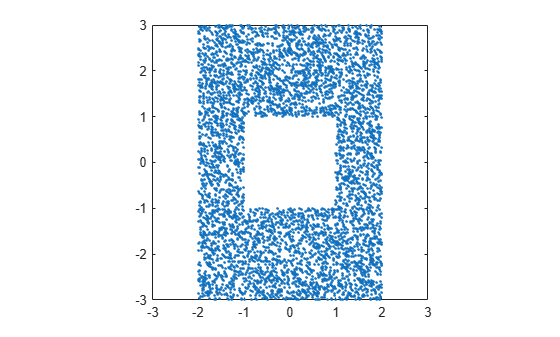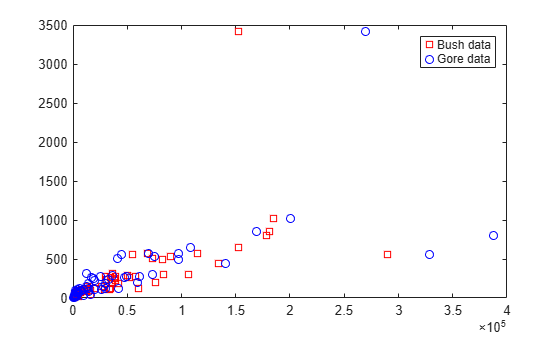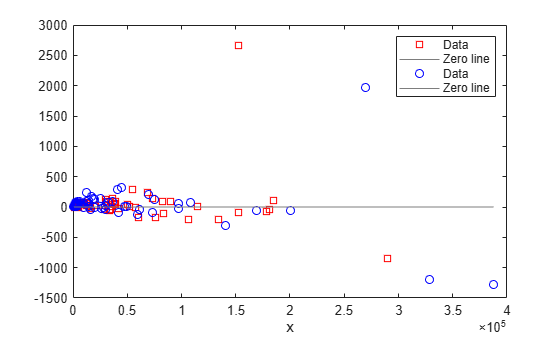excludedata

Exclude data from fit

Description

example

tf = excludedata(x,y,'box',box) returns a logical array that indicates which elements are outside the box in the xy-plane specified by box. The elements of tf equal 1 for data points outside the box and 0 for data points inside the box. To exclude data when fitting a curve using fit, specify tf as the 'Exclude' value.

example

tf = excludedata(x,y,'domain',domain) identifies data points that have x-values outside the interval domain.

example

tf = excludedata(x,y,'range',range) identifies the data points with y-values outside the interval range.

tf = excludedata(x,y,'indices',indices) identifies the data points with indices equal to indices.

Examples

collapse all

Visualize exclusion rules using random data.

Generate random x and y data.

xdata = -3 + 6*rand(1,1e4);
ydata = -3 + 6*rand(1,1e4);

As an example, exclude data that is either inside the box [-1 1 -1 1] or outside the domain [-2 2].

outliers1 = ~excludedata(xdata,ydata,'box',[-1 1 -1 1]);
outliers2 = excludedata(xdata,ydata,'domain',[-2 2]);
outliers = outliers1|outliers2;

Plot the data that is not excluded. The white area corresponds to regions that are excluded.

plot(xdata(~outliers),ydata(~outliers),'.')
axis([-3 3 -3 3])
axis squareLoad the vote counts and county names for the state of Florida from the 2000 U.S. presidential election.

Use the vote counts for the two major party candidates, Bush and Gore, as predictors for the vote counts for the third-party candidate Buchanan, and plot the scatters:

plot(bush,buchanan,'rs')
hold on
plot(gore,buchanan,'bo')
legend('Bush data','Gore data')Assume a model where a fixed proportion of Bush or Gore voters choose to vote for Buchanan.

f = fittype({'x'})
f =
Linear model:
f(a,x) = a*x

Exclude the data from absentee voters, who did not use the controversial “butterfly” ballot.

nobutterfly = strcmp(counties,'Absentee Ballots');

Perform a bisquare weights robust fit of the model to the two data sets, excluding absentee voters.

bushfit = fit(bush,buchanan,f,'Exclude',nobutterfly,'Robust','on');
gorefit = fit(gore,buchanan,f,'Exclude',nobutterfly,'Robust','on');

Robust fits give outliers a low weight, so large residuals from a robust fit can be used to identify the outliers.

figure
plot(bushfit,bush,buchanan,'rs','residuals')
hold on
plot(gorefit,gore,buchanan,'bo','residuals')Calculate the residuals.

bushres = buchanan - feval(bushfit,bush);
goreres = buchanan - feval(gorefit,gore);

Identify large residuals as those outside the range [-500 500].

bushoutliers = excludedata(bush,bushres,'range',[-500 500]);
goreoutliers = excludedata(gore,goreres,'range',[-500 500]);

Display the counties corresponding to the outliers. Miami-Dade and Broward counties correspond to the largest predictor values. Palm Beach county, the only county in the state to use the “butterfly” ballot, corresponds to the largest residual values.

counties(bushoutliers)
ans = 2x1 cell
{'Palm Beach'}

counties(goreoutliers)
ans = 3x1 cell
{'Broward'   }
{'Palm Beach'}

Input Arguments

collapse all

Data sites of data values, specified as a numeric vector.

Data values, specified as a numeric vector.

Box to find data outside of, specified as a numeric vector [xmin xmax ymin ymax] with four elements.

Example: [-1 1 0 2]

Domain to find data outside of, specified as a numeric vector [xmin xmax] with two elements.

Example: [-1 1]

Range to find data outside of, specified as a numeric vector [ymin ymax] with two elements.

Example: [3 4]

Indices of data points to find, specified as a numeric vector.

Example: [3 7 9]